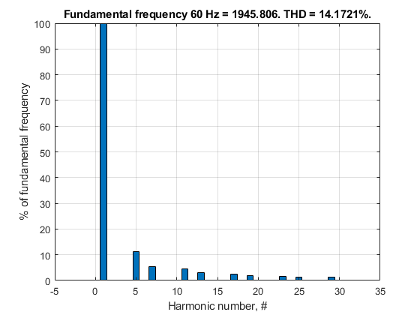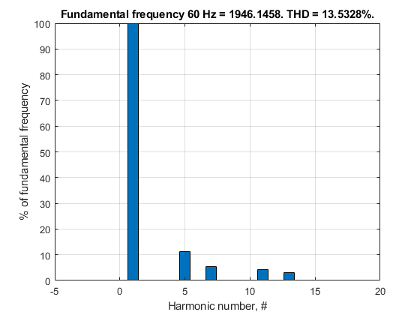# ee_plotHarmonics

Plot percentage of fundamental magnitude versus harmonic order

## Syntax

``ee_plotHarmonics(loggingNode)``
``ee_plotHarmonics(loggingNode,valueIdx)``
``ee_plotHarmonics(loggingNode,valueIdx,tOfInterest)``
``ee_plotHarmonics(loggingNode,valueIdx,tOfInterest,nPeriodOfInterest)``
``````ee_plotHarmonics(loggingNode,valueIdx,tOfInterest,nPeriodOfInterest,... offsetOfInterest)``````
``````ee_plotHarmonics(loggingNode,valueIdx,tOfInterest,nPeriodOfInterest,... offsetOfInterest,nHarmonic)``````

## Description

example

````ee_plotHarmonics(loggingNode)` plots a bar chart of percentage of fundamental magnitude versus harmonic order of the `simscape.logging.Node` of an AC or periodic variable. The title of the bar chart includes the fundamental frequency, fundamental peak value, and total harmonic distortion (THD) percentage.You enter the input arguments in a specific order. The Simscape™ logging node input argument is required. All other input arguments are optional and have default values. If you are specifying a value for a subsequent optional input argument, enter `[]` to use the default value for an optional input argument.The `ee_plotHarmonics` function uses the `ee_getHarmonics` function to: Find the points in the ith signal (valueIdx) where the Simscape log crosses a threshold (offsetOfInterest).Use the crossing points to find the required number of periods (nPeriodOfInterest) preceding the specified time (tOfInterest).Calculate the harmonic magnitudes, up to and including the required number of harmonics (nHarmonic).Input the down-selected data to the Goertzel algorithm, which calculates the harmonic magnitudes up to and including the required number of harmonics (nHarmonic). NoteThe `ee_getHarmonics` function uses threshold crossing points to determine the fundamental frequency of the data. If your input data is noisy or crosses the threshold more frequently than half of the fundamental period, filter it before you use the `ee_plotHarmonics` function to plot it. The `ee_plotHarmonics` function then inputs the harmonic orders and harmonic magnitudes to the `ee_calculateThdPercent` function to calculate the THD.`ee_plotHarmonics(loggingNode,valueIdx)` uses the index into value data.`ee_plotHarmonics(loggingNode,valueIdx,tOfInterest)` uses the simulation time.`ee_plotHarmonics(loggingNode,valueIdx,tOfInterest,nPeriodOfInterest)` uses the number of periods of fundamental frequency.```ee_plotHarmonics(loggingNode,valueIdx,tOfInterest,nPeriodOfInterest,... offsetOfInterest)``` uses the DC offset.```ee_plotHarmonics(loggingNode,valueIdx,tOfInterest,nPeriodOfInterest,... offsetOfInterest,nHarmonic)``` uses the number of harmonics.```

## Examples

### Plot Using Default Values

This set of function arguments uses the Simscape logging node `simlog_SixPulseHarmonics.Sensing_current.Current_Sensor.I`, which contains data from a three-phase current. The function analyzes the default signal, which is the first, or a-phase, signal at the final simulation time. The function uses the default values of 12 for the number of periods of the signal, 0V for the signal bias, and 30 for the number of harmonics.

```openExample("simscapeelectrical/SixPulseHarmonicsExample") sim('SixPulseHarmonics') ee_plotHarmonics(simlog_SixPulseHarmonics.Sensing_current.Current_Sensor.I)```### Plot Using Specified Values

This set of function arguments uses the Simscape logging node `simlog_SixPulseHarmonics.Sensing_current.Current_Sensor.I`, which contains data from a three-phase current. The function analyzes the second, or b-phase, signal at a simulation time of `0.5` s. The function uses `10` periods of the signal, assuming a bias of `1`V. The function analyzes `15` harmonics.

```openExample("simscapeelectrical/SixPulseHarmonicsExample") sim('SixPulseHarmonics') ee_plotHarmonics(simlog_SixPulseHarmonics.Sensing_current.Current_Sensor.I,2,0.5,10,1,15)```### Plot Using Default and Specified Values

This set of function arguments uses the Simscape logging node `simlog_SixPulseHarmonics.Sensing_current.Current_Sensor.I`, which contains data from a three-phase current. The function analyzes the first, or a-phase, signal at a simulation time of `0.5` s. The function uses `12` periods of the signal, assuming a bias of `1`V. The function analyzes the default number, `30`, of harmonics.

```openExample("simscapeelectrical/SixPulseHarmonicsExample") sim('SixPulseHarmonics') ee_plotHarmonics(simlog_SixPulseHarmonics.Sensing_current.Current_Sensor.I,[],0.5,[],1) ```## Input Arguments

collapse all

Simscape logging node, specified as a 1-by-1 `simscape.logging.Node`. You create a `simscape.logging.Node` by running a simulation with Simscape logging enabled. To learn how to enable data logging, see Enable Data Logging for the Whole Model.

Example: `simlog.Load.V`

The Simscape logging node simlog.Load.V contains data from a three-phase voltage.

Index into value data, specified as a scalar. Specifies the ith variable of interest in the Simscape log.

Example: `2`

Specify the b-phase, which is the second signal from a three-phase voltage.

Example: `[]`

Use [] to specify the default value of `1`. The a-phase, which is the first signal from a three-phase voltage, is the default signal of interest.

Data Types: `single` | `double` | `int8` | `int16` | `int32` | `int64` | `uint8` | `uint16` | `uint32` | `uint64`

Simulation time of interest for harmonic analysis, specified as a scalar.

Example: `2.3`

Specify a 2.3s simulation time.

Data Types: `single` | `double` | `int8` | `int16` | `int32` | `int64` | `uint8` | `uint16` | `uint32` | `uint64`

Number of periods of fundamental frequency to be included in harmonic analysis, specified as a scalar.

Example: `10`

Specify `10` periods of the signal.

Data Types: `single` | `double` | `int8` | `int16` | `int32` | `int64` | `uint8` | `uint16` | `uint32` | `uint64`

DC offset in the input signal, specified as a scalar. The function uses this value to find the periods of interest.

Example: `1`

Specify a bias of `1`V for the signal.

Data Types: `single` | `double` | `int8` | `int16` | `int32` | `int64` | `uint8` | `uint16` | `uint32` | `uint64`

Number of harmonics to include in analysis, specified as a scalar.

Example: `15`

Specify that the number of harmonics to be analyzed is `15`.

Data Types: `single` | `double` | `int8` | `int16` | `int32` | `int64` | `uint8` | `uint16` | `uint32` | `uint64`

## Version History

Introduced in R2014a